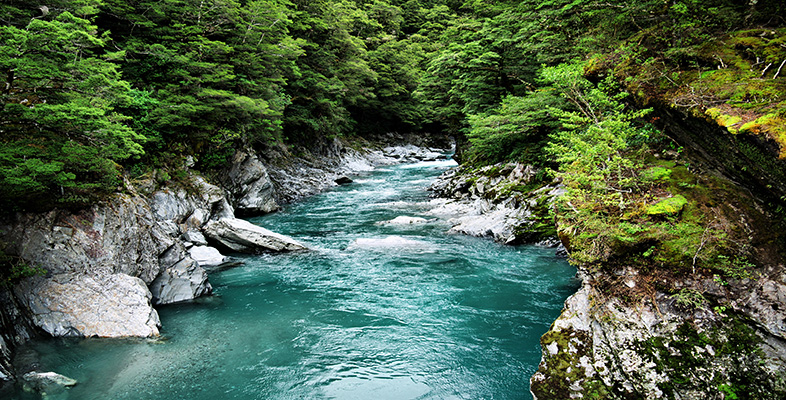Water for life

Start this free course now. Just create an account and sign in. Enrol and complete the course for a free statement of participation or digital badge if available.

Free course

# 6.4 Chemical equations and chemical reactions

The previous section shows how different elements can either exist on their own or combine with other elements to make compounds. This section builds on these ideas by looking at chemical reactions in more detail. It also shows how chemical shorthand can be extended to describing chemical reactions.

First, consider some of the molecules described earlier: water, methane, carbon dioxide and ammonia.

## Question 26

What are the formulas for each of these four molecules?

The formulas are H2O, CH4, CO2 and NH3, respectively.

Although you don't need to remember the chemical formulas of compounds introduced in this course, you'll probably find it useful to memorise a few such as those for the four molecules above, plus those for hydrogen (H2), nitrogen (N2) and oxygen (O2) molecules. This will help you in reading and writing these chemical formulas without continually referring back to previous sections.

Now you will look at reactions involving the elements hydrogen, carbon and oxygen and the compounds methane, water and carbon dioxide.

Hydrogen will react with oxygen when it is ignited (it is quite explosive) to form water. To write such a reaction in terms of a chemical equation, the substances that undergo the reaction are put on the left and the substances that are produced in the reaction are put on the right. The reactants on the left are linked to the products on the right by an arrow.

The equation can be written as a word equation 'hydrogen and oxygen make water' or, using a little chemical shorthand, it can be written as: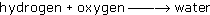where the arrow means 'goes to'.

Now, try using chemical shorthand to write the equation. Substituting symbols in the word equation gives: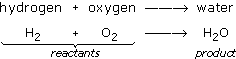The equation shows the reactants on the left of the arrow being converted to the product on the right. However, there is something wrong with this equation. You can see what is wrong by looking at Figure 21 where the reactants and products are shown as a diagram.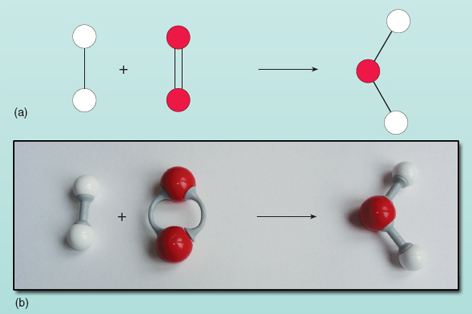Figure 21 The unbalanced equation for the reaction between hydrogen and oxygen to give water: (a) diagrammatic representation; (b) ball-and-stick model. Note that the oxygen molecule comprises two oxygen atoms linked by a double bond. This is because each oxygen atom has two 'hooks' to link up

Counting the numbers of atoms on each side of the equation shows that there are two oxygen atoms on the left compared with one on the right. The number of oxygen atoms on both sides of the equation must be equal - they can't magically appear and disappear during a reaction. It is not possible to change the composition of the water molecule on the right of the equation as the water molecule exists as a group of two hydrogen atoms and one oxygen atom all bonded together. Each oxygen molecule supplies two oxygen atoms so will always form two water molecules, provided there are two hydrogen molecules (each being a unit of two atoms) to react with it. Thus two molecules of hydrogen and one molecule of oxygen are needed to make two molecules of water. The reaction is accurately expressed by:There are now the same numbers of each type of atom on both sides of the equation: the chemical equation is balanced, so we can now replace the arrow with an equals sign.

A final tidy-up to avoid repeating the hydrogen molecules on the left of the equation and the water molecules on the right is to represent them by 2H2 rather than H2 + H2. So the balanced chemical equation becomes: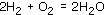This is shown diagrammatically in Figure 22.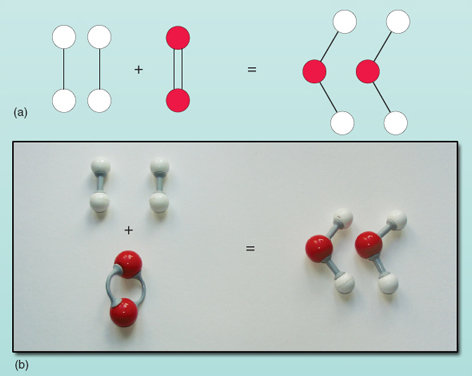Figure 22 The balanced equation for the reaction between hydrogen and oxygen to give water: (a) diagrammatic representation; (b) ball-and-stick model

Chemical equations show in a very concise way not only which atoms and molecules react together to form the products but also how many of each sort of atom and molecule are involved. It is important to remember that the number before a molecule means the number of that particular molecule. For example 3H2O means three molecules of water, giving a total of six hydrogen and three oxygen atoms.

Chemical equations must balance; the number of atoms of each type of element on both sides of the equation must be equal.

You now know how to 'read' or interpret a chemical equation - and how it represents a chemical process. However, chemists are also very adept at writing chemical equations as a form of shorthand to describe various chemical processes or reactions. Writing chemical equations is like writing in any foreign language - it requires practice to become fluent. However, all budding scientists have to start somewhere and the section below shows you how to do this using a very familiar chemical reaction. If you wish to check whether you need to study this section, try Question 20 first.

S154_1